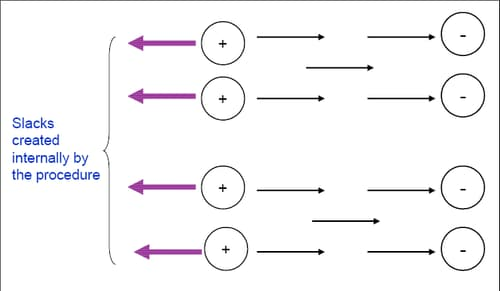### Handling Excess Supply or Demand

The `supdem` value of a node can be specified in the following formats:

• in the NODEDATA= data set, using the `_supdem_` or `_sd_` list variable

• in the ARCDATA= data set, using the `_SUPPLY_` and `_DEMAND_` list variables

If there is only one supply (demand) node, then use the SOURCE= (SINK=) option to refer to it, and use the SUPPLY= (DEMAND=) option to specify its `supdem` value.

To ensure feasibility, there are different methods by which flow can be added to or drained from the network. This extra flow can be added to or drained from the network at either the supply or demand nodes. The new EXCESS= option is used to address such instances.

For pure networks there are two valid values that can be specified for the EXCESS= option: EXCESS=ARCS and EXCESS=SLACKS.

EXCESS=ARCS is the default value. An extra node, referred to as `_EXCESS_`, is added to the network and is connected to the actual network by excess arcs.

• If total supply exceeds total demand, then `_EXCESS_` is an extra demand node with demand equal to total supply minus total demand.

• If the THRUNET option is specified, the excess arcs are directed away from any actual demand node (even nodes with missing D demand) and toward `_EXCESS_`.

• Or else if there are demand nodes with missing D demands, the excess arcs are directed away from these nodes and toward `_EXCESS_`.

• Or else the excess arcs are directed away from the supply nodes and toward `_EXCESS_`.

• If the total demand exceeds the total supply, then `_EXCESS_` is an extra supply node with supply equal to the total demand minus the total supply.

• If the THRUNET option is specified, the excess arcs are directed away from `_EXCESS_` and toward any actual supply node (even nodes with missing S supply.)

• Or else if there are supply nodes with missing S supplies, the excess arcs are directed away from `_EXCESS_` and toward these nodes.

• Or else the excess arcs are directed away from `_EXCESS_` and toward the demand nodes.

The node `_EXCESS_` and the associated arcs are created to ensure that the problem presented to the optimizer has a total supply equal to the total demand. They are neither displayed in the optimal solution nor saved in any output SAS data set.

If EXCESS=SLACKS is specified, then slack variables are created for some flow conservation constraints instead of having the node `_EXCESS_` and excess arcs. The flow conservation constraint (which was an inequality) is now converted to an equality with the addition of the slack variable. Alternatively, you can think of these slacks as arcs with one of their end nodes missing — they are directed from a node but not toward any other node (or directed toward a node but not from any other node). Figure 6.27 presents a clearer picture of this.

Figure 6.27: `EXCESS=SLACKS`, Total Supply Exceeds Total Demand, THRUNET Not Specified, No Nodes with Missing DemandNote: When you specify EXCESS=SLACKS, the interior point solver is used. The output SAS data set needs to be specified by the `CONOUT=` data set, even if side constraints are not present. Also, when you specify the EXCESS=SLACKS option, the size of the model submitted to the optimizer is smaller than with EXCESS=ARCS since it no longer has the `_EXCESS_` node and the excess arcs associated with it.